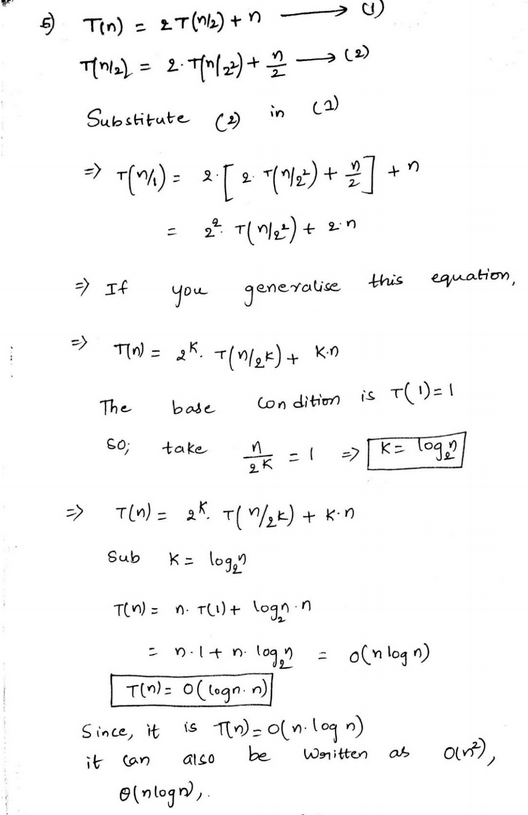GATE | GATE-CS-2005 | Question 37

• Difficulty Level : Medium
• Last Updated : 28 Jun, 2021

Suppose T(n) = 2T (n/2) + n, T(0) = T(1) = 1

Which one of the following is FALSE?
(A) T(n) = O(n2)
(B) T(n) = θ(n log n)
(C) T(n) = Ω(n2)
(D) T(n) = O(n log n)

Explanation:See question 4 of https://www.geeksforgeeks.org/data-structures-and-algorithms-set-23/

This solution is contributed by Anil Saikrishna Devarasetty

Quiz of this Question

My Personal Notes arrow_drop_up
Recommended Articles
Page :